# Polynomials¶

## Polynomial evaluation (polyval)¶

mpmath.polyval(ctx, coeffs, x, derivative=False)

Given coefficients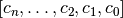and a number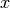, polyval() evaluates the polynomial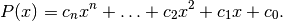If derivative=True is set, polyval() simultaneously evaluates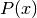with the derivative,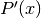, and returns the tuple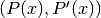.

```>>> from mpmath import *
>>> mp.pretty = True
>>> polyval([3, 0, 2], 0.5)
2.75
>>> polyval([3, 0, 2], 0.5, derivative=True)
(2.75, 3.0)
```

The coefficients and the evaluation point may be any combination of real or complex numbers.

## Polynomial roots (polyroots)¶

mpmath.polyroots(ctx, coeffs, maxsteps=50, cleanup=True, extraprec=10, error=False)

Computes all roots (real or complex) of a given polynomial.

The roots are returned as a sorted list, where real roots appear first followed by complex conjugate roots as adjacent elements. The polynomial should be given as a list of coefficients, in the format used by polyval(). The leading coefficient must be nonzero.

With error=True, polyroots() returns a tuple (roots, err) where err is an estimate of the maximum error among the computed roots.

Examples

Finding the three real roots of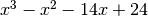:

```>>> from mpmath import *
>>> mp.dps = 15; mp.pretty = True
>>> nprint(polyroots([1,-1,-14,24]), 4)
[-4.0, 2.0, 3.0]
```

Finding the two complex conjugate roots of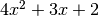, with an error estimate:

```>>> roots, err = polyroots([4,3,2], error=True)
>>> for r in roots:
...     print(r)
...
(-0.375 + 0.59947894041409j)
(-0.375 - 0.59947894041409j)
>>>
>>> err
2.22044604925031e-16
>>>
>>> polyval([4,3,2], roots)
(2.22044604925031e-16 + 0.0j)
>>> polyval([4,3,2], roots)
(2.22044604925031e-16 + 0.0j)
```

The following example computes all the 5th roots of unity; that is, the roots of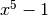:

```>>> mp.dps = 20
>>> for r in polyroots([1, 0, 0, 0, 0, -1]):
...     print(r)
...
1.0
(-0.8090169943749474241 + 0.58778525229247312917j)
(-0.8090169943749474241 - 0.58778525229247312917j)
(0.3090169943749474241 + 0.95105651629515357212j)
(0.3090169943749474241 - 0.95105651629515357212j)
```

Precision and conditioning

The roots are computed to the current working precision accuracy. If this accuracy cannot be achieved in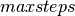steps, then a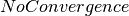exception is raised. The algorithm internally is using the current working precision extended by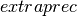. Ifwas raised, that is caused either by not having enough extra precision to achieve convergence (in which case increasingshould fix the problem) or too low(in which case increasingshould fix the problem), or a combination of both.

The user should always do a convergence study with regards toto ensure accurate results. It is possible to get convergence to a wrong answer with too low.

Provided there are no repeated roots, polyroots() can typically compute all roots of an arbitrary polynomial to high precision:

```>>> mp.dps = 60
>>> for r in polyroots([1, 0, -10, 0, 1]):
...     print r
...
-3.14626436994197234232913506571557044551247712918732870123249
-0.317837245195782244725757617296174288373133378433432554879127
0.317837245195782244725757617296174288373133378433432554879127
3.14626436994197234232913506571557044551247712918732870123249
>>>
>>> sqrt(3) + sqrt(2)
3.14626436994197234232913506571557044551247712918732870123249
>>> sqrt(3) - sqrt(2)
0.317837245195782244725757617296174288373133378433432554879127
```

Algorithm

polyroots() implements the Durand-Kerner method , which uses complex arithmetic to locate all roots simultaneously. The Durand-Kerner method can be viewed as approximately performing simultaneous Newton iteration for all the roots. In particular, the convergence to simple roots is quadratic, just like Newton’s method.

Although all roots are internally calculated using complex arithmetic, any root found to have an imaginary part smaller than the estimated numerical error is truncated to a real number (small real parts are also chopped). Real roots are placed first in the returned list, sorted by value. The remaining complex roots are sorted by their real parts so that conjugate roots end up next to each other.

References